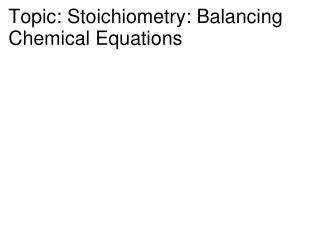DownloadDownload PresentationTopic: Stoichiometry: Balancing Chemical Equations

# Topic: Stoichiometry: Balancing Chemical Equations

Download Presentation## Topic: Stoichiometry: Balancing Chemical Equations

- - - - - - - - - - - - - - - - - - - - - - - - - - - E N D - - - - - - - - - - - - - - - - - - - - - - - - - - -
##### Presentation Transcript

1. Topic: Stoichiometry: Balancing Chemical Equations

2. What is stoichiometry? • The study of quantitative relationships in a balanced chemical equation • Balance equations represent chemical reactions • Remember in chemical reactions 3 things are conserved • Matter • Energy • charge

3. Law of Conservation of Matter • Matter is neither created nor destroyed in a chemical reaction • Mass reactants = Mass products • Chemical bonds in reactants may break • New bonds may form to produce products • Number of atoms of each element is “constant” • # of atoms of each element is the same on both sides of the equation

4. Writing Chemical Equations • Begin with word equation • Solid Tin (IV) Oxide is heated with Hydrogen gas to form tin metal and water vapor. • Next go to skeleton equation: replace names of substances with chemical formulas • SnO2(s) +H2(g) Sn(s) + H2O(g) • Balance skeleton equation: # of atoms of each element is the same on both sides of the equation (law of conversation of mass) - let’s figure out how to balance

5. Equation Balancing…let’s RAPR A PSnO2(s) +H2(g) Sn(s) + H2O(g) Sn O H 1 - - 1 2 - - 1 2 - - 2 NOT BALANCED

6. SnO2(s) + H2(g) Sn(s) + H2O(g) 2 2 Sn O H 1 - - 1 2 - - 1 2 4 2 - - 2 4 • Find the most complex compound and then compare the number of each element on product side and reactant side • Look at H2O – 2 Hydrogen and 1 oxygen • On reactant side, 2 Oxygen and 2 Hydrogen • Use Coefficients to balance the numbers of each atom, check number of atoms as you go: NOTE: NEVER CHANGE SUBSCRIPTS BALANCED 

7. NOTE: Coefficients must be in lowest possible ratios 2SnO2(s) + 4H2(g) 2Sn(s) + 4H2O(g)

8. Fe + O2 Fe2O3 • HINTS: • Most Complex = Fe2O3 • ODDS ARE AKWARD, so Try to make O3 even!!!!!!! 4Fe + 3O2  2 Fe2O3

9. AgNO3 + MgCl2 Mg(NO3)2 + AgCl • HINTS: • Keep polyatomics together • NO3 • Ag • Cl • Mg 2 AgNO3 + MgCl2 Mg(NO3)2 + 2 AgCl

10. Na + H2O  NaOH + H2 2 Na + 2 H2O  2 NaOH + H2 • HINTS: • ODDS ARE AKWARD – try to make even • H on product side H + H2 = 3 hydrogens